FreeBuf.COM网络安全行业门户，每日发布专业的安全资讯、技术剖析。FreeBuf+小程序

2020-12-25 16:37:19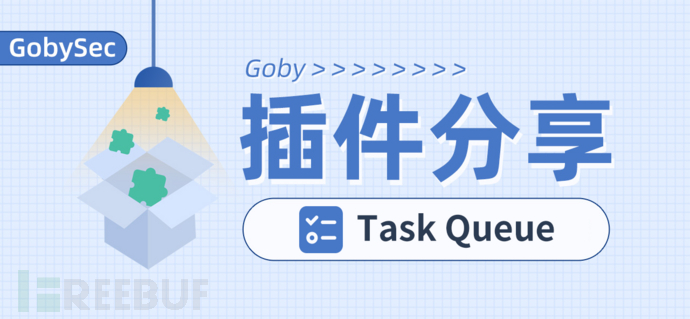## 0x001 插件效果

### 1.1 插件使用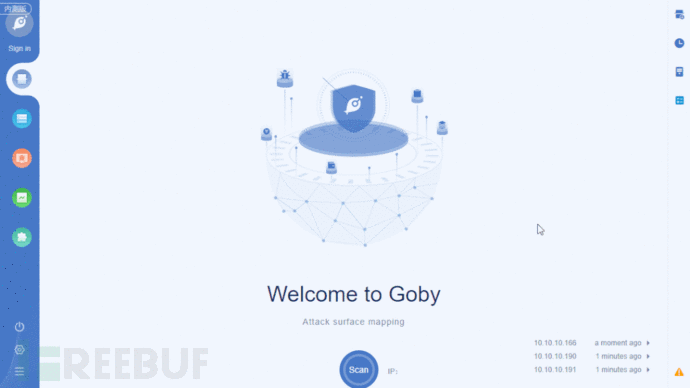### 1.2 插件入口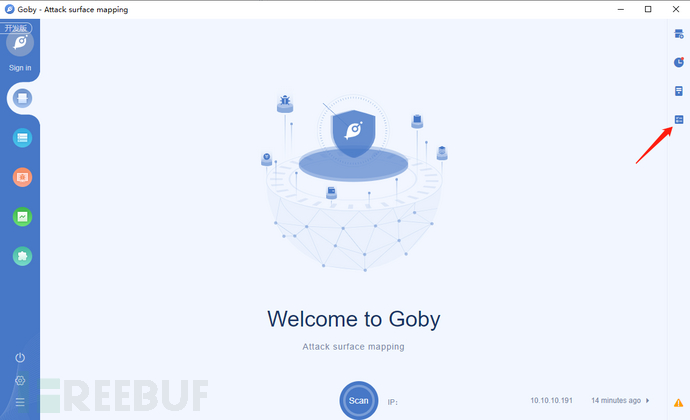### 1.3 操作队列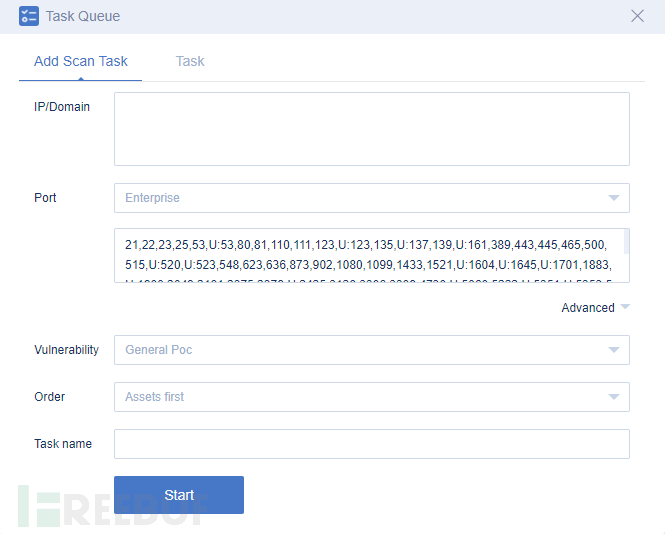Task可以查看队列中的任务详情，还可以对队列中的任务进行修改删除操作。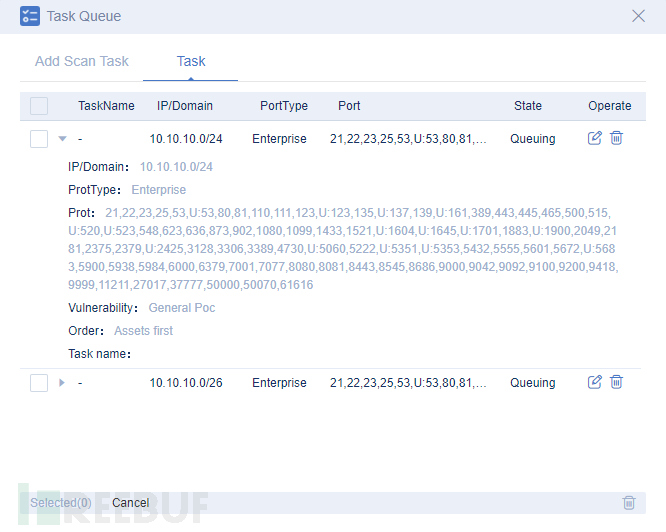## 0x002 插件开发

### 2.1 确定功能的入口点

```"contributes": {
"views": {
"toolbar":[
{
"icon": "src/assets/img/logo2.png",
}
]
}
}```

### 2.2 任务队列数据的存储

`fs.writeFile(this.filePath,JSON.stringify({"data":[]}, null, 6),(err)=>{}`

### 2.3 确定实现功能需要用到的API

2.3.1 获取扫描数据

```goby.getPortList().then((res)=>{
config.port = res.data;
let con = ``;
for(let i in res.data){
con += `
<option value="\${res.data[i].type}">\${res.data[i].type}</option>
`;
}
\$('.port').html(con);
form.render('select');
\$('.portcontent').val(res.data.value);
})
goby.getVulnerabilityList().then((res)=>{
let con = ``;
for(let i in res.data){
con += `
<option value="\${res.data[i]}">\${res.data[i]}</option>
`;
}
\$('.vulnerability').html(con);
form.render('select');
})
goby.getOrderList().then((res)=>{
let con = ``;
for(let i in res.data){
con += `
<option value="\${res.data[i]}">\${res.data[i]}</option>
`;
}
\$('.order').html(con);
form.render('select');
})```

### 2.3.2 绑定事件通知bindEvent

```//因为暂停后会涉及到操作队列文件，所以延迟1秒刷新页面
goby.bindEvent('onPauseScan',(res)=>{
setTimeout(()=>{
init();
},1000)
})```

### 2.3.3 开始扫描startScan

```goby.startScan(queue.data[index]).then((res)=>{
queue.data[index].state = 1;
fs.writeFile(this.filePath,JSON.stringify(queue, null, 6),(err)=>{
if(err){
reject(err);
}else{
resolve(res);
}
})
});```

### 2.4  业务开发

`window.taskQueue = new taskQueue();`

```let goby = parent.goby;

```\$('.con').delegate('.delete','click',function(){
init();
});
})```

## 0x003 小结

https://gobies.org/docs.html

# 工具 # Goby

+ 收入我的专辑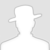• 0 文章数
• 0 评论数
• 0 关注者